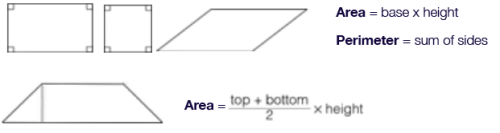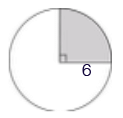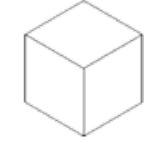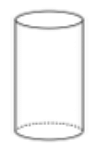# Polygons, Geometry For GMAT Notes | Study Quantitative Aptitude for GMAT - GMAT

## GMAT: Polygons, Geometry For GMAT Notes | Study Quantitative Aptitude for GMAT - GMAT

The document Polygons, Geometry For GMAT Notes | Study Quantitative Aptitude for GMAT - GMAT is a part of the GMAT Course Quantitative Aptitude for GMAT.
All you need of GMAT at this link: GMAT

GEOMETRY

Polygons

Polygons include any shape with 4 or more sides and usually involve calculating an area or perimeter.Circles

ALL circle questions come down to the RADIUS. With the radius, you can solve almost any question. When a circle interacts with another shape, pay careful attention to how the radius factors into that other shape.

Area = πr2
circumference = 2πr
Sector Area: A fraction of the area
Arc Length: A fraction of the circumferenceExample:
Area = 36π
Sector Shaded (90° is a quarter of the circle) = 9π
Circumference =  12π
Arc (90° carves a quarter of the circumference) = 3π

Multi-Shape

These shapes will always vary. Nevertheless, they are always combinations of the other GMAT geometry rules, and therefore rarely contains any unique rules. Break this shapes apart by looking for shapes you’re familiar with.

Note: Multi-Shape generally show up 1 time on Test Day, can take 3 minutes to solve, and tend to be worth very little (and sometimes nothing). Your best move on these questions is typically to TRIAGE.

Solids

Solid questions involve rectangular solids (boxes), cubes (a type of rectangular solid in which all dimensions are the same) and cylinders (cans or tubes). They usually involve volume (what the solid will “hold”) and sometimes surface area.Volume = base x width x height
Surface Area (Sum of the faces) =  Sum of: 2(base x width) 2(width x height) 2(base x height)Volume = πr2h
Surface Area (top + bottom + side) =  2πr2 + 2πr

The document Polygons, Geometry For GMAT Notes | Study Quantitative Aptitude for GMAT - GMAT is a part of the GMAT Course Quantitative Aptitude for GMAT.
All you need of GMAT at this link: GMATUse Code STAYHOME200 and get INR 200 additional OFF

## Quantitative Aptitude for GMAT

75 videos|87 docs|156 tests

Track your progress, build streaks, highlight & save important lessons and more!

,

,

,

,

,

,

,

,

,

,

,

,

,

,

,

,

,

,

,

,

,

,

,

,

;